Request a Free Counselling Session from our Expert Mentor# NCERT Solution for Class Xth Maths Chapter 1 Real Numbers

This chapter is the foundation of all mathematics as it deals with the fundamental concepts of numbers, including rational and irrational numbers, prime numbers, and composite numbers. It is essential to have a clear understanding of these concepts to understand and solve more advanced mathematical problems.
In this chapter, you will learn about Euclid’s division algorithm, the fundamental theorem of arithmetic, and the properties of real numbers such as commutativity, associativity, and distributivity. You will also learn about the decimal expansion of real numbers, and how to differentiate between rational and irrational numbers.
This chapter has a total of 4 exercises, which cover various concepts related to real numbers. The NCERT Solutions for Class Xth Maths Chapter 1 – Real Numbers provide step-by-step solutions to all the exercises, which will help you to understand the concepts and solve the problems effectively. These solutions are designed to be easy to understand and follow, helping you to build a strong foundation in mathematics.
Overall, this chapter is an essential part of the mathematics curriculum and will lay the groundwork for more advanced mathematical topics.

## Answers of Class Xth Maths Chapter 1 Real Numbers

Unit 1

Real Numbers

Exercise 1.1

Q1 :

Use Euclid’s division algorithm to find the HCF of:

(i) 135 and 225

Since 225 > 135.,

We apply the division lemma to 225 and 135

Since remainder 90 ≠ 0, we apply the division lemma to 135 and 90 to obtain

We consider the new divisor 90 and new remainder 45, and apply the division lemma to obtain

Since the remainder is zero, the process stops. Since the divisor at this stage is 45,

Therefore, the HCF of 135 and 225 is 45.

(ii) 196 and 38220

Since 38220 > 196,

we apply the division lemma to 38220 and 196

Since the remainder is zero, the process stops. Since the divisor at this stage is 196, Therefore, HCF of 196 and 38220 is 196.

(iii) 867 and 255

Since 867 > 255,

We apply the division lemma to 867 and 255

Since remainder 102 ≠ 0, we apply the division lemma to 255 and 102

We consider the new divisor 102 and new remainder 51, and apply the division lemma to obtain

Since the remainder is zero, the process stops.

Since the divisor at this stage is 51, Therefore, HCF of 867 and 255 is 51.

Q2 :

Show that any positive odd integer is of the form   where q is some integer.

Let a be any positive integer and b = 6.

Then, by Euclid’s algorithm,

a = 6q + r

For some integer q and r = 0, 1, 2, 3, 4, 5 because 0 ≤ r < 6.

Therefore,

Also,

where is a positive integer

where is an integer

where is an integer

Clearly,

are of the form 2k + 1, where k is an integer.

Therefore, are not exactly divisible by 2. Hence, these expressions of numbers are odd numbers.

And therefore, any odd integer can be expressed in the form

Q3 :

An army contingent of 616 members is to march behind an army band of 32 members in a parade. The two groups are to march in the same number of columns. What is the maximum number of columns in which they can march?

HCF (616, 32) will give the maximum number of columns in which they can march. We can use Euclid’s algorithm to find the HCF.

The HCF (616, 32) is 8.

Therefore, they can march in 8 columns each.

Q4 :

Use Euclid’s division lemma to show that the square of any positive integer is either of form 3m or 3m + 1 for some integer m.

Let a be any positive integer and b = 3. Then a = 3q + r for some integer q ≥ 0 and r = 0, 1, 2 because

Or,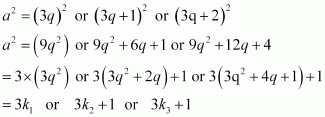Where are some positive integers

Hence, it can be said that the square of any positive integer is either of the form 3m or 3m + 1.

Q5 :

Use Euclid’s division lemma to show that the cube of any positive integer is of the form 9m, 9m + 1 or 9m +8.

Let a be any positive integer and b = 3

a = 3q + r, where q ≥ 0 and 0 ≤ r < 3

Therefore, every number can be represented as these three forms.

There are three cases.

Case 1: When a = 3q,

Where m is an integer such that m

Case 2: When a = 3q + 1,

Where m is an integer such that

Case 3: When a = 3q + 2,

Where m is an integer such that Therefore, the cube of any positive integer is of the form

Exercise 1.2

Q1:

Express each number as product of its prime factors:

Q2 :

Find the LCM and HCF of the following pairs of integers and verify that LCM × HCF = product of the two numbers.

Hence, product of two numbers = HCF × LCM

Hence, product of two numbers = HCF × LCM

Hence, product of two numbers = HCF × LCM

Q3 :

Find the LCM and HCF of the following integers by applying the prime factorization method.

*Q4 :

Given that HCF (306, 657) = 9, find LCM (306, 657).

Q5 :

Check whether 6n can end with the digit 0 for any natural number n.

If any number ends with the digit 0, it should be divisible by 10 or in other words, it will also be divisible by 2 and 5 as

Prime factorization of

It can be observed that 5 is not in the prime factorization of 6n. Hence, for any value of n, 6n will not be divisible by 5.

Therefore, 6n cannot end with the digit 0 for any natural number n.

Q6 :

Explain why are composite numbers.

Numbers are of two types – prime and composite. Prime numbers can be divided by 1 and only itself, whereas composite numbers have factors other than 1 and itself.

It can be observed that

The given expression has 6 and 13 as its factors. Therefore, it is a composite number.

1009 cannot be factorized further. Therefore, the given expression has 5 and 1009 as its factors. Hence, it is a composite number.

*Q7 :

There is a circular path around a sports field. Sonia takes 18 minutes to drive one round of the field, while Ravi takes 12 minutes for the same. Suppose they both start at the same point and at the same time, and go in the same direction. After how many minutes will they meet again at the starting point?

It can be observed that Ravi takes lesser time than Sonia for completing 1 round of the

circular path. As they are going in the same direction, they will meet again at the same time when Ravi will have completed 1 round of that circular path with respect to Sonia.

And the total time taken for completing this 1 round of circular path will be the LCM of time taken by Sonia and Ravi for completing 1 round of circular path respectively i.e., LCM of 18 minutes and 12 minutes.

Therefore, Ravi and Sonia will meet together at the starting point after 36 minutes.

Exercise 1.3

Q1 :

Prove that   is irrational.

Let  is a rational number.

Therefore, we can find two integers a, b (b ≠ 0) such that

Let a and b have a common factor other than 1. Then we can divide them by the common factor, and assume that a and bare co-prime.

Therefore, a2is divisible by 5  and it can be said that a is divisible by . Let a = 5k, where k is an integer

This means that b2is divisible by 5 and hence, b is divisible by . This implies that a and b have 5 as a common factor.

And this is a contradiction to the fact that a and b are co-prime. Hence,  cannot be

expressed as  or it can be said that is irrational.

Q2 :

Prove that  is irrational.

Let  is rational.

Therefore, we can find two integers a, b (b ≠ 0) such that

Since a and b are integers,  will also be rational and therefore, is rational.

This contradicts the fact that   is irrational. Hence, our assumption that is rational is false. Therefore,    is irrational.

Q3 :

Prove that the following are irrationals:

Let  is rational.

Therefore, we can find two integers a, b (b ≠ 0) such that

is rational as a and b are integers.

Therefore,  is rational which contradicts to the fact that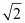is irrational.

Hence, our assumption is false and is irrational.

Let   is rational.

Therefore, we can find two integers a, b (b ≠ 0) such that for some integers a &b

is rational as a and b are integers. Therefore,   should be rational.

This contradicts the fact that is irrational.

Therefore, our assumption that is rational is false. Hence,  is irrational.

Let    be rational.

Therefore, we can find two integers a, b (b ≠ 0) such that

Since a and b are integers,  is alsorational and hence,should be rational. This contradicts the fact that is irrational. Therefore, our assumption is false and hence,

Exercise 1.4

Q1 :

Without actually performing the long division, state whether the following rational numbers will have a terminating decimal expansion or a non-terminating repeating decimal expansion:

(i)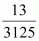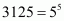The denominator is of the form.

Hence, the decimal expansion of is terminating.

(ii)

The denominator is of the form

Hence, the decimal expansion of is terminating.

(iii)

Since the denominator is not in the form and it also contains 7 and 13 as its factors, its decimal expansion will be non-terminating repeating.

(iv)

The denominator is of the form

Hence, the decimal expansion of  is terminating.

(v)

Since the denominator is not in the form and it has 7 as its factor, the decimal expansion of is non-terminating repeating.

(vi)

The denominator is of the form

Hence, the decimal expansion of is terminating.

(vii)

Since the denominator is not of the form , and it also has 7 as its factor, the decimal expansion of    is non-terminating repeating.

(viii)

The denominator is of the form 5n.

Hence, the decimal expansion of  is terminating.

(ix)

The denominator is of the form .

Q2 :

Write down the decimal expansions of those rational numbers in Question 1 above which have terminating decimal expansions.

(i)

(ii)

(viii)

*Q3:

The following real numbers have decimal expansions as given below. In each case, decide whether they are rational or not. If they are rational, and of the form what can you say about the prime factor of q?

(i) 43.123456789 (ii) 0.120120012000120000… (iii)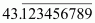(i) 43.123456789

Since this number has a terminating decimal expansion, it is a rational number of the form  and q is of the form

i.e., the prime factors of q will be either 2 or 5 or both.

(ii) 0.120120012000120000 …

The decimal expansion is neither terminating nor recurring. Therefore, the given number is an irrational number.

(iii)Since the decimal expansion is non-terminating recurring, the given number is a rational number of the form

and q is not of the form   i.e., the prime factors of q will also have a factor other than 2 or 5.

## NCERT Solutions for Class 10 Maths Chapter 1- Real Numbers

Real Number is one of the important topics in Maths as it has a weightage of 6 marks in class 10 (Number system – Real Numbers) Maths board exams. The average number of questions asked from this chapter is usually 3. Three questions were asked from this chapter in the previous year board examination (2018).

1. One out of three questions in part A (1 marks).
2. One out of three questions in part B (2 marks).
3. One out of three questions in part C (3 marks).

• Euclid’s Division Algorithm
• The Fundamental Theorem of Arithmetic
• Revisiting Rational & Irrational Numbers
• Decimal Expansions

List of Exercises in class 10 Maths Chapter 1:

Exercise 1.1 Solutions 5 Question ( 4 long, 1 short)
Exercise 1.2 Solutions 7 Question ( 4 long, 3 short)
Exercise 1.3 Solutions 3 Question ( 3 short)
Exercise 1.4 Solutions 3 Question ( 3 short)

Real Numbers is introduced in Class 9 and this is discussed more in details in Class 10. NCERT Solutions for Class 10 Maths Chapter 1 Real Numbers provides the answers for the questions present in this chapter. The chapter discusses the real numbers and their applications. The divisibility of integers using Euclid’s division algorithm says that any positive integer a can be divided by another positive integer b such that the remainder will be smaller than b. On the other hand, The Fundamental Theorem of Arithmetic works on multiplication of positive integers.

The chapter starts with the introduction of real numbers in section 1.1 followed by two very important topics in section 1.2 and 1.3

• Euclid’s Division Algorithm – It includes 5 questions based on Theorem 1.1 – Euclid’s Division Lemma.
• The Fundamental Theorem of Arithmetic – Explore the applications of this topic which talks about the multiplication of positive integers, through solutions of the 7 problems in Exercise 1.2.

Next, it discusses the following topics which were introduced in class 9.

• Revisiting Rational & Irrational Numbers – In this, the solutions for 3 problems in Exercise 1.3 are given which also use the topic in the last Exercise 1.2.
• Decimal Expansions – It explores when the decimal expansion of a rational number is terminating and when it is recurring. It includes a total of 3 problems with sub-parts in Exercise 1.4

### Key Features of NCERT Solutions for Class 10 Maths Chapter 1- Real Numbers

• These NCERT Solutions let you solve and revise the whole syllabus of class 10.
• After going through the stepwise solutions given by our subject expert teachers, you will be able to score more marks.
• It follows NCERT guidelines which help in preparing the students accordingly.
• It contains all the important questions from the examination point of view.
• It helps in scoring well in maths in exams.

Conclusion

The NCERT Solutions for Class Xth Maths Chapter 1 – Real Numbers provide a comprehensive understanding of the fundamental concepts of numbers, including rational and irrational numbers, prime numbers, and composite numbers. The chapter covers important topics such as Euclid’s division algorithm, the fundamental theorem of arithmetic, and the properties of real numbers.
The solutions provided by the NCERT are well-structured and easy to understand, with step-by-step explanations for all the exercises. The solutions are designed to help students develop their problem-solving skills and gain a deeper understanding of the concepts covered in the chapter.
Overall, the NCERT Solutions for Class Xth Maths Chapter 1 – Real Numbers are an excellent resource for students who want to excel in the subject. The solutions will not only help them score well in their exams but also prepare them for more advanced topics in mathematics. It is crucial to have a clear understanding of the concepts covered in this chapter as it forms the foundation for all mathematical concepts.

### What are the main topics covered in the NCERT Solutions for Class 10 Maths Chapter 1?

The main topics covered in the NCERT Solutions for Class 10 Maths Real Numbers are Euclid’s division algorithm, the fundamental theorem of arithmetic, revisiting rational & irrational numbers and decimal expansions. Based on these concepts, our experts created the solutions effectively. By giving importance to these concepts students can score good marks in class tests as well as in Class 10 board exams.

### How many exercises are there in NCERT Solutions for Class 10 Maths Chapter 1?

There are four exercises in the NCERT Solutions for Class 10 Maths Real Numbers. These Solutions of NCERT Maths Class 10 Chapter 1 help the students in solving the problems adroitly and efficiently. They also focus on cracking the Solutions of Maths in such a way that it is easy for the students to understand.

### Are NCERT Solutions for Class 10 Maths Chapter 1 important from the exam point of view?

Yes, the first chapter Real Numbers of NCERT Solutions for Class 10 is important from an exam perspective. It contains short answer questions as well as long answer questions to increase the ability of problem-solving. These solutions are created by the SWC’s expert faculty to help students in the preparation of their examinations.